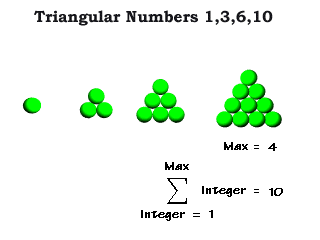## Monday, January 08, 2007

### A Gnu Math Proof

To prove:

cubocta(f) == 10*f*f + 2 for f>0, or 1 if f==0.

We will derive Fuller's formula from first geometric principles, knowing that (f+1)**2 balls in 6 square faces plus (f+1)*(f+2)//2 balls in 8 triangular faces, minus derived amounts for doubly counted edge-only balls, quadruply counted vertex balls, should simplify to our reliable result.

Proof:

Six Square Faces:
6*(f+1)**2 == 6*(f**2 + 2*f + 1) == 6*f**2 + 12*f + 6

Eight Triangular Faces:
8*(f+1)*(f+2)//2 == 4*f**2 + 12*f + 8

Summing these two: 10*f**2 + 24*f + 14

Subtract 1x Edge (but not Vertex) balls:
10*f**2 + 24*f + 14 - 24*(f-1) == 10*f**2 + 38

Subtract 3x Vertex balls:
10*f**2 + 38 - 36 == 10*f**2 + 2.

QED.

The geometer H.S.M. Coxeter stated this proof'd be within range of high schoolers, if not sooner, and I think he's obviously right about that.

We care about this sequence because it models a growing CCP packing, a kind of holodeck we use for a lot of visualizations in science and engineering. It also naturally occurs (we know this empirically).

Notationally, it's possible to wire up the growing and/or shrinking cuboctahedron (variable frequency) to a volume number of 20*f**3 i.e. 20 where f==1.

There's not much proof needed to show the efficacy of this aesthetic design, which I use in my P4E and HP4E curriculum writings quite consistently.